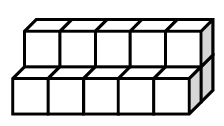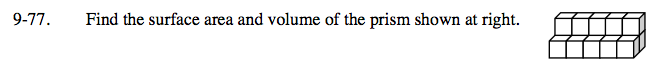Home > CC2MN > Chapter cc29 > Lesson cc29.2.3 > Problem9-77

9-77.Find the surface area and volume of the prism shown at right. Homework Help ✎If each edge measures 1 unit, then:
1) each side surface has an area of 1 un2.
2) the volume of one cube is 1 un3.

How many cubes make up this object?
How many sides make up the surface of the object?

Surface Area = 46 square units
Volume = 15 cubic units